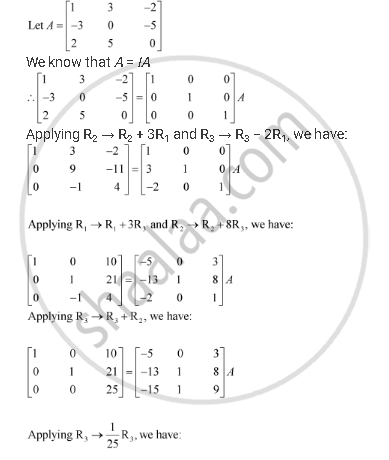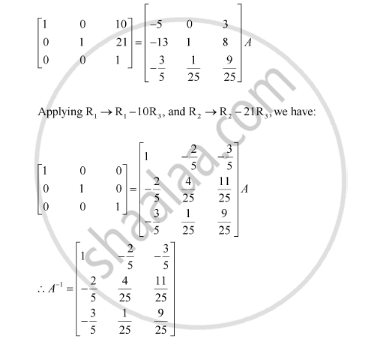Share

# Find the Inverse of Each of the Matrices, If It Exists. [(1,3,-2),(-3,0,-5),(2,5,0)] - CBSE (Science) Class 12 - Mathematics

#### Question

Find the inverse of each of the matrices, if it exists.

[(1,3,-2),(-3,0,-5),(2,5,0)]

#### SolutionIs there an error in this question or solution?

#### Video TutorialsVIEW ALL 

Solution Find the Inverse of Each of the Matrices, If It Exists. [(1,3,-2),(-3,0,-5),(2,5,0)] Concept: Invertible Matrices.
S